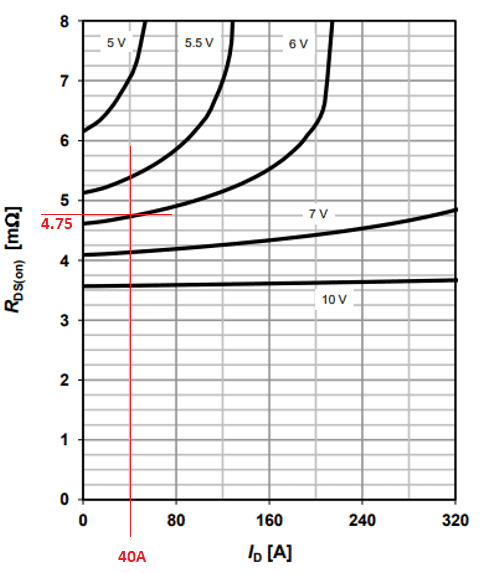# How to Get MOSFET Correct RDSon Value

How to get MOSFET correct RDSon value is the pre-requisite to compute the MOSFET conduction loss or static power dissipation. RDSon is the drain to source on-state resistance of the MOSFET. The term “correct” is relative to the target result. Supposing the target is to compute the maximum power dissipation, then the correct RDSon value must be the worst-case value. On the other hand, if the target is to derive the realistic system efficiency, then the correct RDSon value must be the typical value. Read the article How to Compute MOSFET Conduction Loss to appreciate the application of the correct RDSon value.

## How to Get MOSFET Correct RDSon Value from the Datasheet

The RDSon value is provided in the datasheet. To be more realistic here, let us consider IPP040N06N MOSFET from Infineon Technologies. Infineon is offering great solutions for your designs and products. Visit them at https://www.infineon.com/.

### Aiming for Maximum RDSon

In the datasheet, under Product Summary, there is a specified maximum RDSon value (see below).

Considering our objective is how to get MOSFET correct RDSon value for worst case application, like to derive the maximum static power dissipation or conduction loss, is this the value that we are looking for?

RDSon is a MOSFET complicated parameter. Let us explore further the datasheet. In “6 Typ. drain-source on resistance”, there is a graph of drain current (ID) versus RDSon with different VGS values. The curve values are derived from a typical junction temperature of 25’C.

From the graph, the highest RDSon is happening at the lowest VGS (VGS is the voltage applied in the gate and source terminals). Therefore, the Product Summary value is not yet the maximum value of the RDSon.

Let us assume here (for the sake of explaining clearly on how to get MOSFET correct RDSon) an applied VGS to the MOSFET circuit of 6V with a +/-1V swing. This means that the VGS could go as low as 5V. With this, the worst case RDSon should be derived from the 5V curve.

Since the RDSon is a function of the drain current, there is a need to get the drain current. Let us assume that the drain current is 40A, and from the 5V curve, the corresponding RDSon is 7 milliohms. Therefore, 7 milliohms must be the maximum RDSon that we should be using.

Furthermore, RDSon will vary with temperature. Under “9 Drain-source on-state resistance”, RDSon is plotted to a graph with the junction temperature. Though the graph is plotted with ID = 80A and VGS = 10V, still it is a good reference for discussion on how RDSon affected with temperature. If you have access to the curve that corresponds for VGS = 5V and drain current of 40A, then you can further see the increase of the RDSon value at a certain temperature above 25’C. For example, the worst-case junction temperature could reach 100’C (this will be happening at the maximum ambient temperatures), then get the RDSon at 100’C.

## How to Get MOSFET Correct RDSon Value for Typical Application

Typical RDSon value as discussed is the preferred to get the realistic system efficiency. In our example above, the VGS is 6V with a swing of +/-1V. Since this time, we are looking for the typical value of RDson, let us only consider the 6V since this is the typical level of the VGS. So, from “6 Typ. drain-source on resistance”, the corresponding RDSon at the drain current of 40A is 4.75 milliohm.

Moreover, for typical RDson, it is important to get the equivalent typical junction temperature. This happens at the typical ambient temperature. The typical ambient temperature is usually 25’C. This value will give an equivalent junction temperature as the junction temperature is a function of the actual power dissipation plus the ambient temperature. Supposing the equivalent typical junction temperature is 30’C, look from the graph (TJ versus RDSon) the equivalent RDSon.

## Summary

These are the key takeaways regarding how to get MOSFET correct RDSon value.

1. RDSon value could be worst-case or typical.

2. Worst case RDSon value could be used for calculating the maximum static power dissipation.

3. Typical RDSon is more preferrable to use in getting the system efficiency.

4. RDSon is a function of drain current.

5. RDSon is also a function of temperature.

6. In computing the worst case RDSon value, the minimum VGS must be considered as well as the maximum junction temperature.

This site uses Akismet to reduce spam. Learn how your comment data is processed.